## Point-Point Distance--3-D

The Line Element is(1)

so the Arc Length between the pointsandis(2)

and the quantity we are minimizing is(3)

Finding the derivatives gives(4)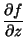(5)

and(6)(7)

so the Euler-Lagrange Differential Equations become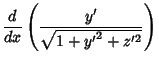(8)(9)

These give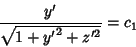(10)(11)

Taking the ratio,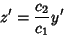(12)(13)(14)

which gives(15)(16)

Therefore,and, so the solution is(17)

which is the parametric representation of a straight line with parameter. Verifying the Arc Length gives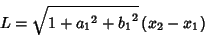(18)

where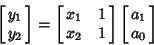(19)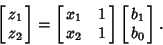(20)